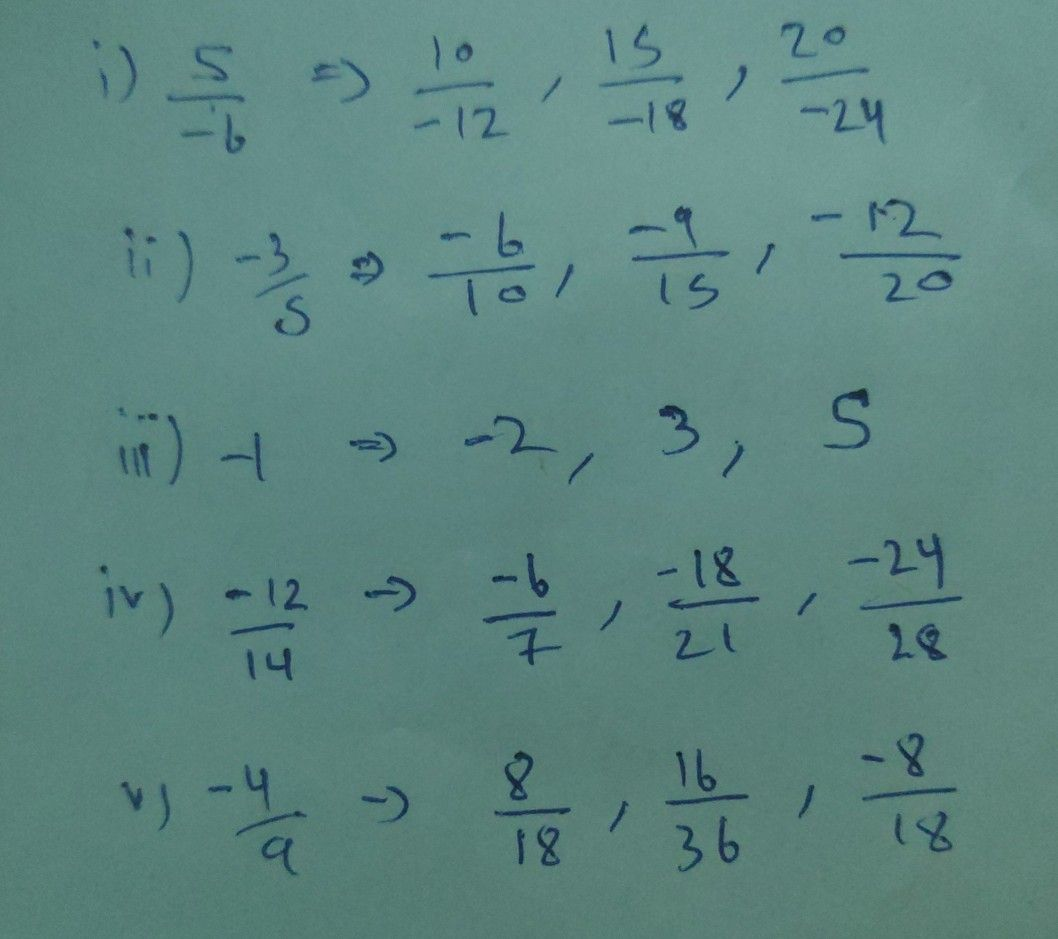Symbol
Problem$7$ Find three equivalent rational numbers of each of the following rational numbers: $\left(11$ $\dfrac {5} {-6}$ $111^{\right)}$ $\dfrac {-3} {5}$ $10$ $1$ (iv) $\dfrac {-12} {14}$ $\left(y\dfrac {-4} {9}$ A Which o o